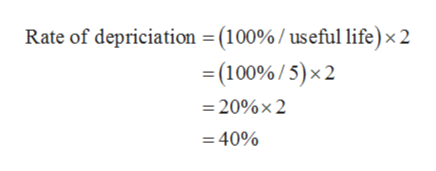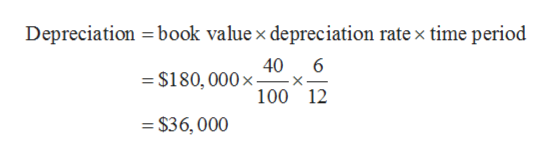# Pearson Company bought a machine on July 1, 2022. The machine cost \$180,000 and had an expected salvage value of \$0. The life of the machine was estimated to be 5 years. Pearson uses a December 31 year end.  The depreciation expense for 2023 using the double declining balance method of depreciation isGroup of answer choices\$57,600\$36,000\$30,000\$50,600

Question
12 views

Pearson Company bought a machine on July 1, 2022. The machine cost \$180,000 and had an expected salvage value of \$0. The life of the machine was estimated to be 5 years. Pearson uses a December 31 year end.  The depreciation expense for 2023 using the double declining balance method of depreciation is

\$57,600
\$36,000
\$30,000
\$50,600
check_circle

Step 1

Depreciation refers to the amount of permanent loss in the value of an asset. It is reduction in the value of an asset which can be due to natural calamity, wear n tear, handling issues.

Under double-declining-balance method, the depreciation is charged at double the rate of depreciation calculated under straight-line method.

Step 2

Cost of the machine: \$180,000

Useful life: 5 years

Rate of depreciation under double-declining-balance method is calculated as follows:help_outlineImage TranscriptioncloseRate of depriciation =(100%/ useful life)× 2 = (100% / 5)× 2 = 20%× 2 = 40% fullscreen
Step 3

Depreciation on machine for year 2022:

Cost of machine: \$180,000

Time period: 6 mont...help_outlineImage TranscriptioncloseDepreciation = book value x depreciation rate x time period 40 6 = \$180, 000× 100 12 = \$36, 000 fullscreen

### Want to see the full answer?

See Solution

#### Want to see this answer and more?

Solutions are written by subject experts who are available 24/7. Questions are typically answered within 1 hour.*

See Solution
*Response times may vary by subject and question.
Tagged in